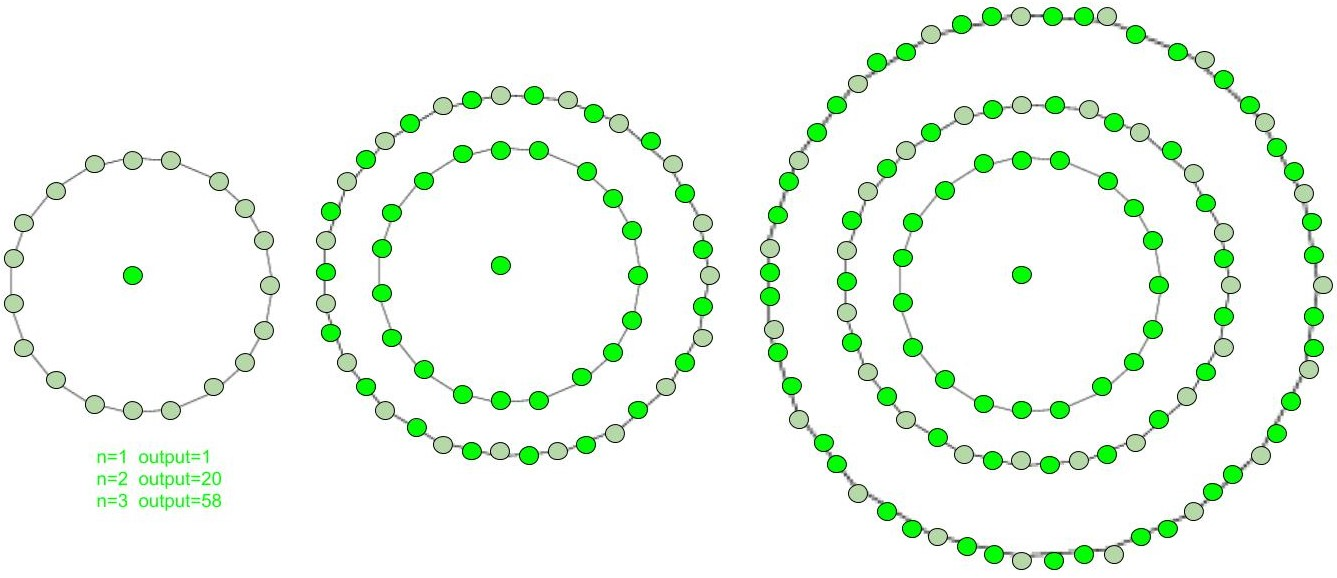Given a number n, find the nth Centered Nonadecagonal number.

A Centered Nonadecagonal Number represents a dot in the centre and other dots surrounding it in successive nonadecagon(19 sided polygon) layers.The first few Centered Nonadecagonal numbers are:
1, 20, 58, 115, 191, 286, 400, 533, 685, 856, 1046, 1255……………………………

Examples :

```Input :  3
Output : 58

Input : 13
Output :1483
```

## Recommended: Please try your approach on {IDE} first, before moving on to the solution.

In mathematics, Centered nonadecagonal number for n-th term is given by :

``````

Below is the basic implementation of the above idea:

## C++

 `// C++ Program to find ` `// nth centered ` `// nonadecagonal number ` `#include ` `using` `namespace` `std; ` ` `  `// centered nonadecagonal ` `//  function ` `int` `center_nonadecagon_num( ``long` `int` `n ) ` `{ ` `    ``// Formula to calculate nth ` `    ``// centered nonadecagonal number ` `    ``return` `(19 * n * n - 19 * n + 2) / 2; ` `} ` ` `  `// Driver Code ` `int` `main() ` `{ ` `    ``long` `int` `n = 2; ` `    ``cout << n << ``"th centered nonadecagonal number : "`  `                   ``<< center_nonadecagon_num(n); ` `    ``cout << endl; ` `    ``n = 7; ` `    ``cout << n << ``"th centered nonadecagonal number : "` `                  ``<< center_nonadecagon_num(n); ` ` `  `    ``return` `0; ` `} `

## Java

 `// Java Program to find nth centered ` `// nonadecagonal number ` `import` `java.io.*; ` ` `  `class` `GFG { ` `     `  `    ``// centered nonadecagonal ` `    ``// function ` `    ``static` `int` `center_nonadecagon_num(``int` `n) ` `    ``{ ` `        ``// Formula to calculate nth ` `        ``// centered nonadecagonal number ` `        ``return` `(``19` `* n * n - ``19` `* n + ``2``) / ``2``; ` `    ``} ` `     `  `    ``// Driver code ` `    ``public` `static` `void` `main (String[] args) ` `    ``{ ` `        ``int` `n = ``2``; ` `        ``System.out.print ( n + ``"th centered "` `                ``+ ``"nonadecagonal number : "``); ` `        ``System.out.println (  ` `                   ``center_nonadecagon_num(n)); ` `     `  `        ``n = ``7``; ` `        ``System.out.print ( n + ``"th centered "` `                ``+ ``"nonadecagonal number : "``); ` `        ``System.out.println( ` `                  ``center_nonadecagon_num(n)); ` `    ``} ` `} ` ` `  `// This code is contributed by m_kit `

## Python3

 `# Program to find nth ` `# centered nonadecagonal number ` `def` `center_nonadecagon_num(n) : ` `     `  `    ``# Formula to calculate  ` `    ``# nth centered nonadecagonal  ` `    ``# number & return it into  ` `    ``# main function. ` `     `  `    ``return` `(``19` `*` `n ``*` `n ``-`  `            ``19` `*` `n ``+` `2``) ``/``/` `2` ` `  `# Driver Code ` `if` `__name__ ``=``=` `'__main__'` `: ` `         `  `    ``n ``=` `2` `    ``print``(n,``"nd centered nonadecagonal "` `+`  `                              ``"number : "``,  ` `                ``center_nonadecagon_num(n)) ` ` `  `    ``n ``=` `7` `    ``print``(n,``"nd centered nonadecagonal "` `+`  `                              ``"number : "``,  ` `                ``center_nonadecagon_num(n)) ` ` `  `# This code is contributed by ajit `

## C#

 `// C# Program to find  ` `// nth centered  ` `// nonadecagonal number ` `using` `System; ` ` `  `class` `GFG ` `{ ` `     `  `    ``// centered nonadecagonal ` `    ``// function ` `    ``static` `int` `center_nonadecagon_num(``int` `n) ` `    ``{ ` `        ``// Formula to calculate nth ` `        ``// centered nonadecagonal number ` `        ``return` `(19 * n * n -  ` `                ``19 * n + 2) / 2; ` `    ``} ` `     `  `    ``// Driver code ` `    ``static` `public` `void` `Main () ` `    ``{ ` `         `  `        ``int` `n = 2; ` `        ``Console.Write ( n + ``"th centered "` `+  ` `                 ``"nonadecagonal number : "``); ` `        ``Console.WriteLine( ` `                 ``center_nonadecagon_num(n)); ` `     `  `        ``n = 7; ` `        ``Console.Write( n + ``"th centered "` `+  ` `                ``"nonadecagonal number : "``); ` `        ``Console.WriteLine( ` `                ``center_nonadecagon_num(n)); ` `    ``} ` `} ` ` `  `// This code is contributed by ajit `

## PHP

 ` `

Output :

```2th centered nonadecagonal number : 20
7th centered nonadecagonal numbe : 400
```

References:
http://oeis.org/A069132

Attention reader! Don’t stop learning now. Get hold of all the important DSA concepts with the DSA Self Paced Course at a student-friendly price and become industry ready.

My Personal Notes arrow_drop_upCheck out this Author's contributed articles.

If you like GeeksforGeeks and would like to contribute, you can also write an article using contribute.geeksforgeeks.org or mail your article to contribute@geeksforgeeks.org. See your article appearing on the GeeksforGeeks main page and help other Geeks.

Please Improve this article if you find anything incorrect by clicking on the "Improve Article" button below.

Improved By : jit_t, ManasChhabra2